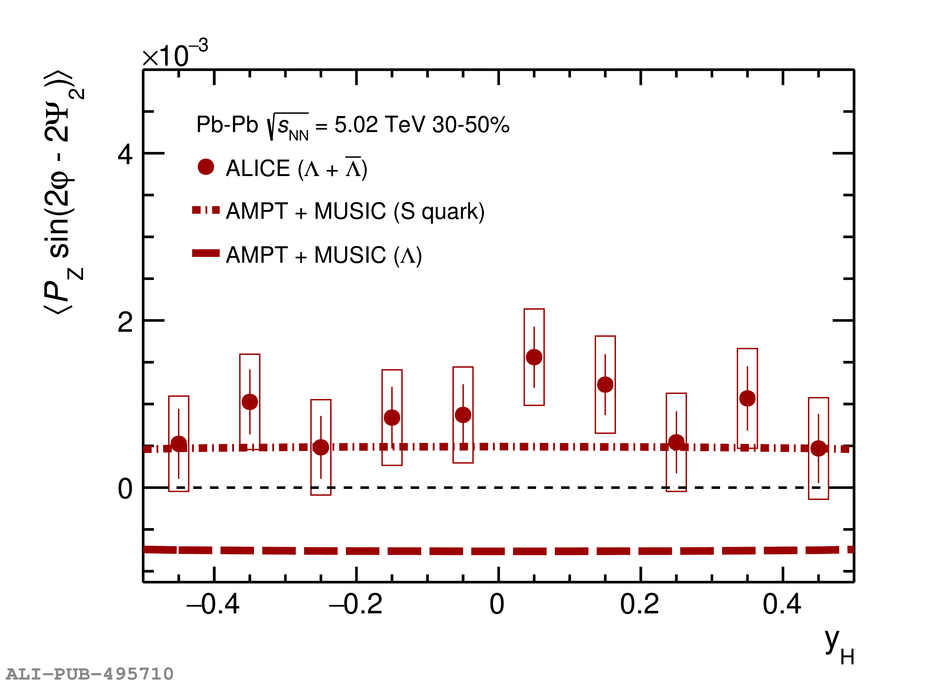# Figure 4

 The rapidity dependence of $\mean{P_{\rm z} \sin(2\varphi - 2\psitwo)}$ averaged for \lam and $\overline\Lambda$ in Pb--Pb collisions at $\sqrt{s_{\rm NN}} =$ 5.02 TeV in semi-central collisions. The model calculations~ for \lam and strange quark for Pb--Pb collisions at $\sqrt{s_{\rm NN}} =$ 5.02 in the 30--50\% centrality interval using the approach described in Ref.~ are shown by dashed-dotted lines.